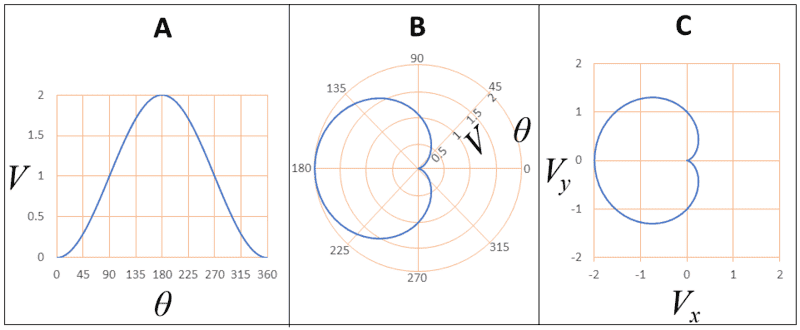# Cartesian and polar terminology

• I
Gold MemberI have a scalar quantity ##V## (let's call it a voltage for concreteness) that is a function of angle ##\theta##. There are two obvious ways to plot it, as a Cartesian plot (see A above) or as a polar plot (see B). I can also express the polar plot in terms of Cartesian coordinates ##V_x = V \, \cos \theta## and ##V_y = V \, \sin \theta## (see C). A particular mathematical process that I have to document involves calculating ##V_x## and ##V_y## as an intermediate step to providing a final output of ##V## and ##\theta##.

(The plots above are illustrative and do not show the function that I have to document in my real-world problem.)

In my document, there is a part where I can describe all this in detail using equations and plots, but there are other parts of the document where I have to summarise the steps of the process in words only. I want to find words to describe A, B, C in just a few words per plot, but still clearly and unambiguously. I have no hesitation in describing B as a "polar plot", but A and C are more problematic. Both could be described as "Cartesian plots" but that isn't enough to distinguish them. Indeed my co-author has unintentionally described both A and C identically, including the phrase "in Cartesian coordinates". I don't really like to describe ##(V,\theta)## as Cartesian coordinates even in the context of Plot A.

As I see it, A and B show the same coordinates but different plots, whereas B and C show the same plot with different coordinates.

Part of my difficulty is that I'm not sure whether the words "polar" and "Cartesian" (or "rectangular" if you prefer) are properties of the coordinates, or of the plots, or the combination of both.

For clarification, ##\theta## does represent a geometrical angle in the real world, but ##V## does not represent a distance.

My suggested terminology is

A = directional voltage response as a Cartesian plot

B = directional voltage response as a polar plot

C = ??

##(V,\theta)## = polar coordinates (or polar voltage coordinates??)

##(V_x,V_y)## = ??

Any thoughts?

#### Attachments

kuruman
Homework Helper
Gold Member
2021 Award
I would call,
A: Plot of V as a function of θ.
B: Polar plot of V.
C: Parametric plot of V.

Such are the names that Mathematica uses for these plots, so there must be some common understanding that that's what they are.

•phinds and mfb
Gold Member
I would call,
A: Plot of V as a function of θ.
B: Polar plot of V.
C: Parametric plot of V.

Such are the names that Mathematica uses for these plots, so there must be some common understanding that that's what they are.

I tried to simplify the statement of my problem to exclude unnecessary detail. But I now realise I've oversimplified it.

Actually I'm not plotting ##V## against ##\theta##. I'm really plotting a third variable ##Q## which is a function of both ##V## and ##\theta##. It's plotted by colouring each pixel on the plot by a colour that represents the value of ##Q##.

kuruman
Homework Helper
Gold Member
2021 Award
Actually I'm not plotting VV against θ\theta. I'm really plotting a third variable Q which is a function of both V and θ. It's plotted by colouring each pixel on the plot by a colour that represents the value of Q.
In that case, I would call that a Contour Plot (another term used by Mathematica) where a given color represents a constant value of Q.

Gold Member
In that case, I would call that a Contour Plot (another term used by Mathematica) where a given color represents a constant value of Q.
But all three are contour plots of ##Q## (as a function of ##V## and ##\theta## in A and B, and of ##V_x## and ##V_y## in C). But I need to find descriptions in words only, no algebraic symbols.

kuruman
Homework Helper
Gold Member
2021 Award
A, B and C in your example, post #1, are not contour plots but show single contours. A contour plot has many contours labeled by numbers as, say isobars on a weather map or different colors as you described in post #3 for values of quantity Q. Perhaps it might be expedient to post the actual plots instead of describing them.

Gold Member
A, B and C in your example, post #1, are not contour plots but show single contours. A contour plot has many contours labeled by numbers as, say isobars on a weather map or different colors as you described in post #3 for values of quantity Q. Perhaps it might be expedient to post the actual plots instead of describing them.
Consider that the curve I drew in my three plots shows one of many contours. Imagine a family of similar "parallel" curves above/around it. I can't show the actual plots.

kuruman